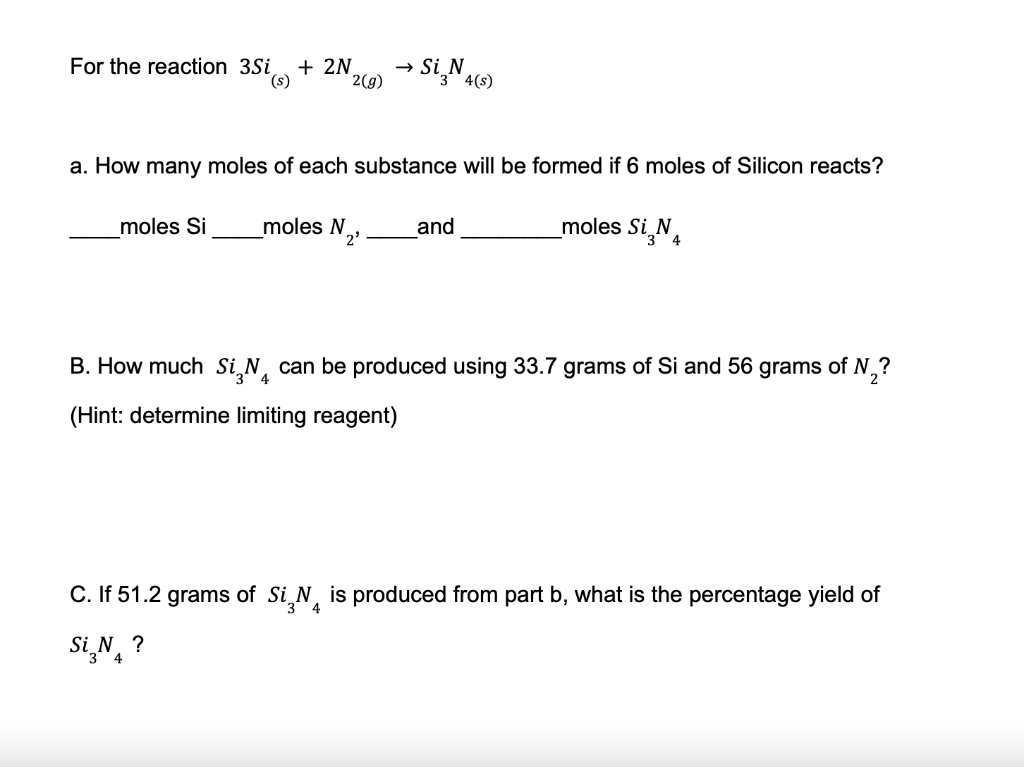Home / Expert Answers / Chemistry / for-the-reaction-3si-s-2n2-g-si3n4-s-a-how-many-moles-of-each-substance-will-be-pa378

# (Solved): For the reaction 3Si(s)+2N2(g)Si3N4(s) a. How many moles of each substance will be ...For the reaction a. How many moles of each substance will be formed if 6 moles of Silicon reacts? moles and moles B. How much can be produced using 33.7 grams of and 56 grams of ? (Hint: determine limiting reagent) C. If of is produced from part b, what is the percentage yield of ?

We have an Answer from Expert

We have the following chemical reaction

a.

'6' moles of silicon are reacting

From the balanced chemical reaction, we see that

'3' moles of   reacts with '2' moles of

Thus '1' mole of   reacts with    moles of

Hence '6' moles of   will react with    moles of

Similarly

'3' moles of    forms '1' moles of

'Hence '6' moles of   will form    moles of
We have an Answer from Expert